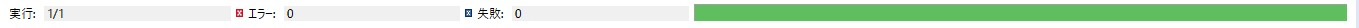# How to access Java Private methods and fields

I left Java for about two weeks, mainly because I wasn't happy with it, but I'm in a hurry for development, so I'm going to test it with JUnit! Even if you try to use a method or field variable whose access modifier is Private, you cannot call it from another class as a matter of course (^ ω ^) ...

But well, I did a lot of research and found out, so I'll make a note of it. If you like, try fertilizing it.

usage environment: OS:Windows 10 java ver：8 IDE:eclipse 4.6 Neon

Save it in a nice local directory.

## Let's add a Jar file

Start eclipse, right-click on [Package Explorer], and add an external Jar from [Build Path]-> [Build Path Configuration]-> [java Build Path].### By the way, what is the API specification like?

Hmmmm.

I don't know at all.

Tell me Guguru Sensei!

## Move in a mess

Prepare a test program. First of all, from the program to be tested.

#### `Sample.java`

``````
public class Sample {

//private field variable
private int x = 10;

//Private and static field variables
private static int y = 20;

//private method
private String testMethod(String x){

return x;
}

//private and static methods
private static String testStaticMethod(String y, String z){

return y + z;
}

}
``````

Next, prepare a class file for JUnit.

#### `SampleTest.java`

``````
import static org.junit.Assert.*;

import org.junit.Test;

import junitx.util.PrivateAccessor;

public class SampleTest {

@Test
/**
*Test function to access private fields and methods
*/
public void privateGetField() throws Throwable {
Sample sample = new Sample();

try {

//A method for accessing private field variables.
//First argument:Instance variable of the class under test Second argument:Field variable name you want to access
int x = (int)PrivateAccessor.getField(sample, "x");

//Compare measured value and expected value by assertion
assertEquals(x,10);

//Private and static field variables can be accessed in a similar way. Explanation omitted
int y = (int)PrivateAccessor.getField(sample, "y");
assertEquals(y,20);

//A method for accessing a private function.
//First argument:Instance variable of the class under test Second argument:Field function name you want to access
//Third argument:Array of argument types Fourth argument:Array of argument values
String method_x = (String)PrivateAccessor.invoke(	sample,
"testMethod",
new Class[]{String.class},
new Object[]{"private_x"});
assertEquals(method_x,"private_x");

//Private and static functions can be accessed in a similar way. Devise the third and fourth arguments according to the number of argument types
String method_y = (String)PrivateAccessor.invoke(	sample,
"testStaticMethod",
new Class[]{String.class,String.class},
new Object[]{"private_","static_y"});
assertEquals(method_y,"private_static_y");

} catch (NoSuchFieldException e) {

e.printStackTrace();

}finally{

}
}
}
``````

## Run

Doya!did it!

### bonus

You can also set a value to a private field variable.

#### `SampleTest.java`

``````

//Omitted on the way

@Test
/**
*A function that sets a value in a private field variable
*/
public void privateSetField(){

Sample sample = new Sample();

try {

//First argument: Instance variable of the class under test Second argument: Name of variable
//Third argument:Value you want to set
PrivateAccessor.setField(sample, "x", 100);

//Check if it was set!
int get_x = (int)PrivateAccessor.getField(sample, "x");
assertEquals(get_x,100);

} catch (NoSuchFieldException e) {

e.printStackTrace();

}finally{

}
}

``````

The end